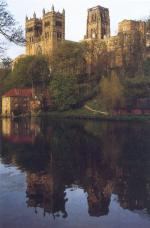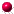Home Outline Key Speakers Participants Photo Poster Programme Talks TopicsEPSRC - London Mathematical Society Durham Symposium Geometry and Arithmetic of Lattices Monday 4th July -- Thursday 14th July 2011
 Online talks: Speaker Title PDF/PPT WMV WMV Abels The coarse geometry of arithmetic quotients, a question of SiegelBaba Graftings and complex projective structuresBergeron Some consequences of Arthurs work on the topology of hyperbolic manifoldsCartwright Enumerating the fake projective planes, part 1Cartwright Enumerating the fake projective planes, part 2Deraux Non-arithmetic lattices in complex hyperbolic geometry, part 1Emery Covolumes of non-uniform arithmetic lattices in PU(n, 1)Gelander On the growth of Betti numbers of arithmetic groupsGoldman Geometric structures on manifolds, part 1Goldman Geometric structures on manifolds, part 2Goldman Geometric structures on manifolds, part 3Gorodnik Large sieve for arithmetic groupsGrossi Constructing (complex) hyperbolic manifoldsGusevskii A characterization of R-Fuchsian groups acting on the complex hyperbolic planeKellerhals Hyperbolic orbifolds of small volumeKlingler Rigidity for complex hyperbolic latticesLeuzinger A geometric characterization of arithmetic Fuchsian groupsLubotzky Counting arithmetic subgroups, surfaces and manifolds, part 1Lubotzky Counting arithmetic subgroups, surfaces and manifolds, part 2Markovic Immersing nearly geodesic surfaces in hyperbolic manifolds and applications, part 1Markovic Immersing nearly geodesic surfaces in hyperbolic manifolds and applications, part 2Millson The geometric theta correspondence and totally geodesic cyclesNikolov Local convergence of congruence subgroups in an arithmetic latticePanov Building complex manifolds using hyperbolic geometryPaulin Representation of integers by product of linear forms and diagonal flowsPaupert Non-arithmetic lattices in complex hyperbolic geometry, part 2Prasad Volumes of arithmetic quotients of semi-simple groups, finiteness theorems, and fake projective spaces, part 1Prasad Volumes of arithmetic quotients of semi-simple groups, finiteness theorems, and fake projective spaces, part 2Raghunathan The first Betti number of compact manifolds of constant curvature, part 1Raghunathan The first Betti number of compact manifolds of constant curvature, part 2Raghunathan The first Betti number of compact manifolds of constant curvature, part 3Rahm Implications of hyperbolic geometry to operator K-theory of arithmetic groupsRapinchuk Weakly commensurable arithmetic groups and length-commensurable locally symmetric spacesRatcliffe Right-angled Coxeter polytopes, hyperbolic 6-manifolds, and a problem of SiegelRemy Quasi-isometry classes of twin building latticesSalehi-Golsefidy Counting lattices in a simple Lie groupSengun On the integral homology of Kleinian groupsStover Arithmeticity and complex reflection groupsThompson Deformed non-equilateral triangle groupsToledo Periods of cubic surfacesVenkataramana On Zariski dense discrete subgroups of SLn (R)Will Teichmuller slices in the PU(2, 1) representation variety of surface groupsProblem Session